## Problem statement

2520 is the smallest number that can be divided by each of the numbers from 1 to 10 without any remainder.

What is the smallest positive number that is evenly divisible by all of the numbers from 1 to 20?

## Thoughts

This is really easy, it doesn't even need programming. The smallest number that can be divided by all numbers in a range is the product of the highest numbers in the range that are integer powers of prime numbers.

## Solution

In case I didn't word my thoughts very well, here is how 2520 is achieved:

•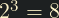•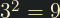•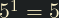•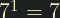•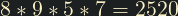We can do the same for the numbers 1-20. The only numbers that decompose into the same prime more than once are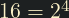and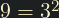``````#include <stdio.h>
int main()
{
printf("%d", 16 * 9 * 5 * 7 * 11 * 13 * 17 * 19);
}
``````